# 如何选择合适的损失函数，请看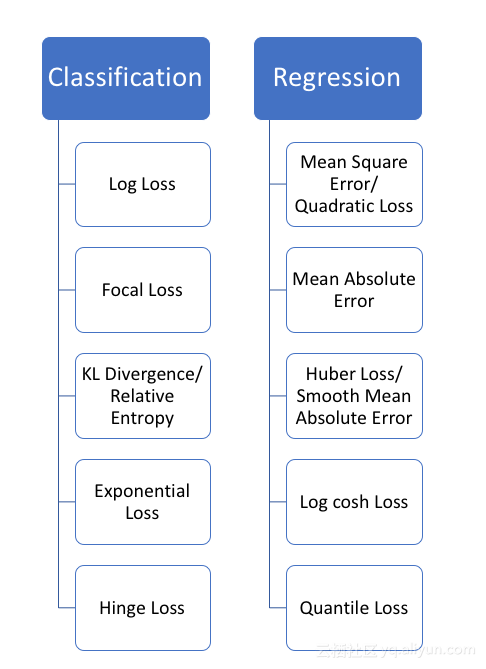﻿﻿

▌回归损失

1、均方误差，二次损失，L2损失（Mean Square Error, Quadratic Loss, L2 Loss）

﻿﻿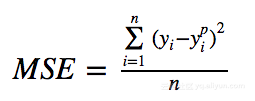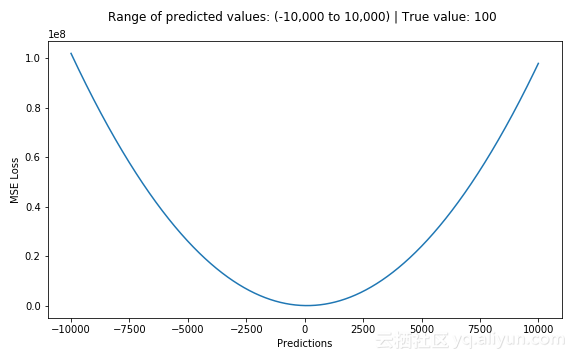﻿﻿
MSE损失（Y轴）与预测值（X轴）关系图

2、平均绝对误差，L1损失（Mean Absolute Error, L1 Loss）

﻿﻿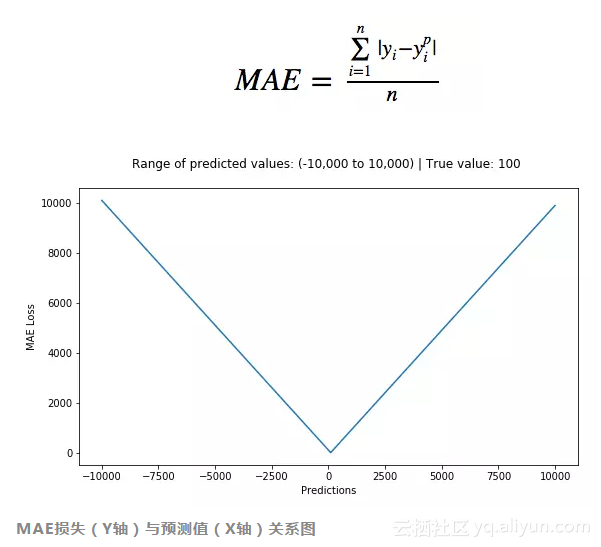3、MSE vs MAE （L2损失 vs L1损失）

＃true：真正的目标变量数组
＃pred：预测数组

def mse(true, pred):
return np.sum(((true – pred)**2))

def mae(true, pred):
return np.sum(np.abs(true – pred))

＃也可以在sklearn中使用

from sklearn.metrics import mean_squared_error
from sklearn.metrics import mean_absolute_error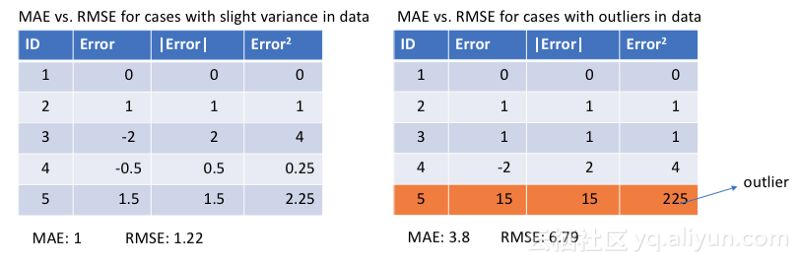﻿﻿

﻿

MAE损失适用于训练数据被离群点损坏的时候（即，在训练数据而非测试数据中，我们错误地获得了不切实际的过大正值或负值）。

﻿﻿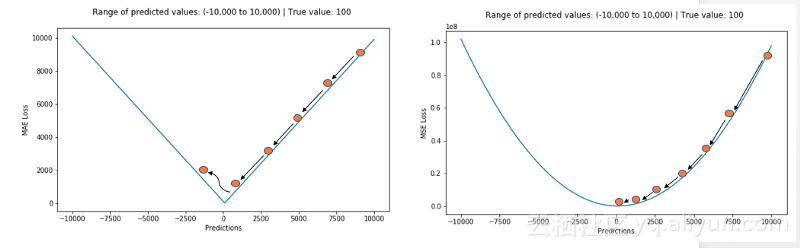L1损失对异常值更加稳健，但其导数并不连续，因此求解效率很低。L2损失对异常值敏感，但给出了更稳定的闭式解（closed form solution）（通过将其导数设置为0）

3、Huber Loss，平滑的平均绝对误差

Huber Loss对数据离群点的敏感度低于平方误差损失。它在0处也可导。基本上它是绝对误差，当误差很小时，误差是二次形式的。误差何时需要变成二次形式取决于一个超参数，(delta)，该超参数可以进行微调。当 𝛿 ~ 0时， Huber Loss接近MAE，当 𝛿 ~ ∞（很大的数）时，Huber Loss接近MSE。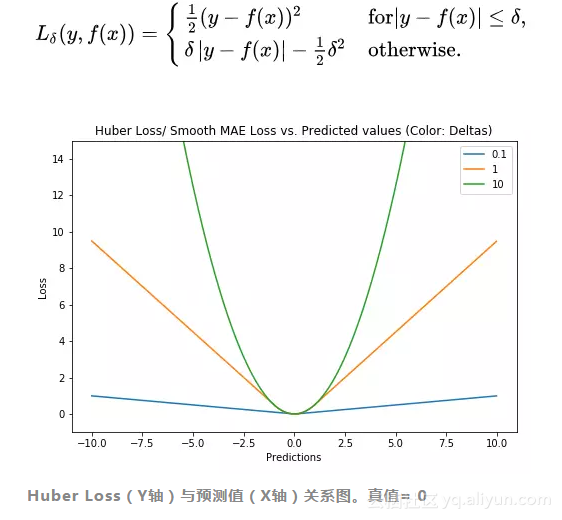delta的选择非常重要，因为它决定了你认为什么数据是离群点。大于delta的残差用L1最小化（对较大的离群点较不敏感），而小于delta的残差则可以“很合适地”用L2最小化。

4、Log-Cosh Loss

Log-cosh是用于回归任务的另一种损失函数，它比L2更加平滑。Log-cosh是预测误差的双曲余弦的对数。

﻿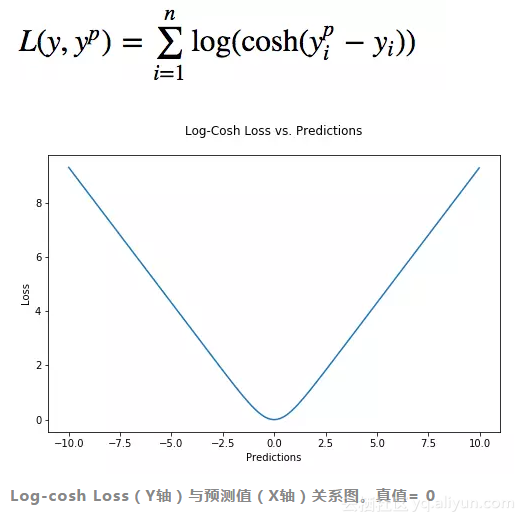﻿﻿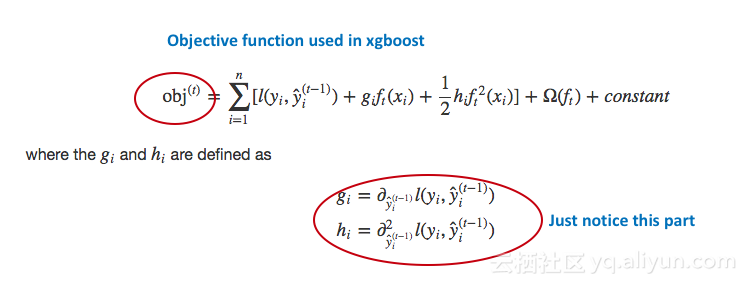XGBoost中使用的目标函数。注意其对一阶和二阶导数的依赖性。

Huber和Log-cosh损失函数的Python代码：

def sm_mae(true, pred, delta):
"""
true: array of true values
pred: array of predicted values

returns: smoothed mean absolute error loss
"""
loss = np.where(np.abs(true-pred) < delta , 0.5*((true-pred)**2), delta*np.abs(true - pred) - 0.5*(delta**2))
return np.sum(loss)

def logcosh(true, pred):
loss = np.log(np.cosh(pred - true))
return np.sum(loss)


5、Quantile Loss（分位数损失）

Quantile 回归 vs 普通最小二乘（Ordinary Least Square, OLS）回归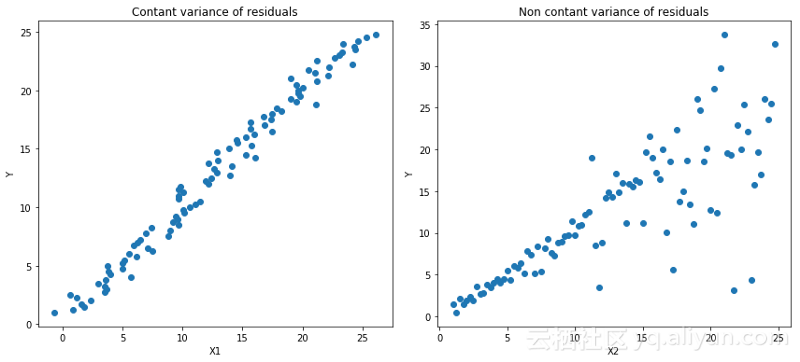﻿﻿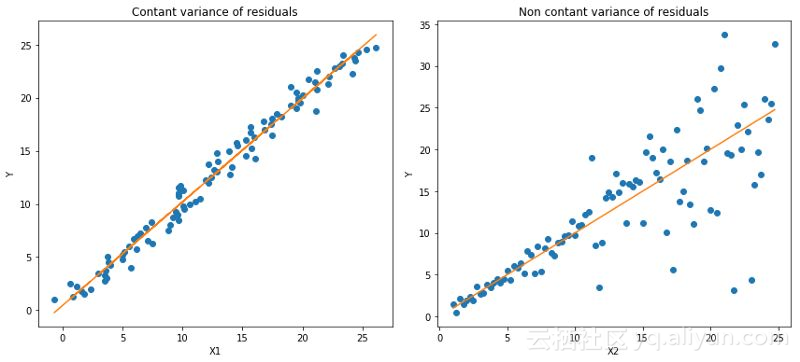﻿﻿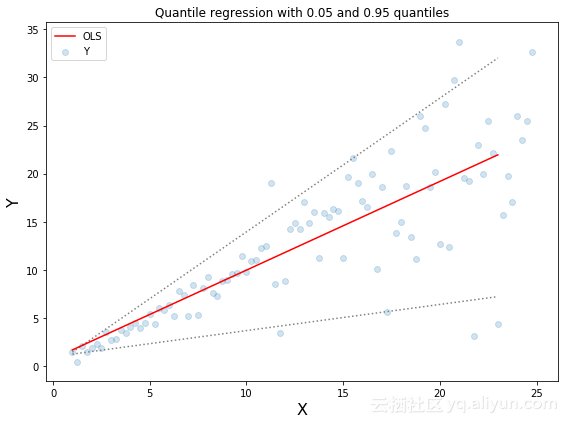﻿﻿
Quantile回归：虚线表示基于0.05和0.95 分位数损失函数的回归估计

Quantile Loss的思想是根据我们是打算给正误差还是负误差更多的值来选择分位数数值。损失函数根据所选quantile (γ)的值对高估和低估的预测值给予不同的惩罚值。举个例子，γ= 0.25的Quantile Loss函数给高估的预测值更多的惩罚，并试图使预测值略低于中位数。

﻿﻿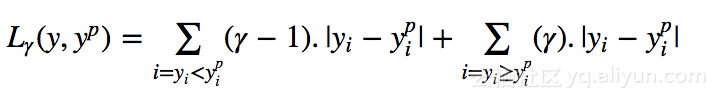γ 是给定的分位数，其值介于0和1之间。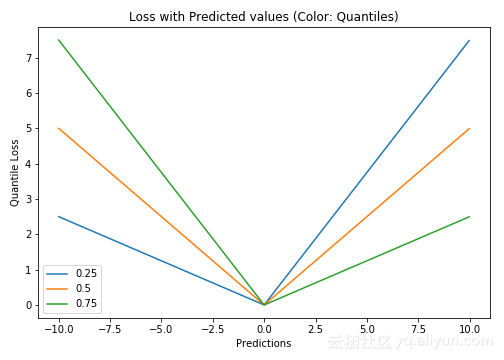Quantile Loss（Y轴）与预测值（X轴）关系图。真值为Y= 0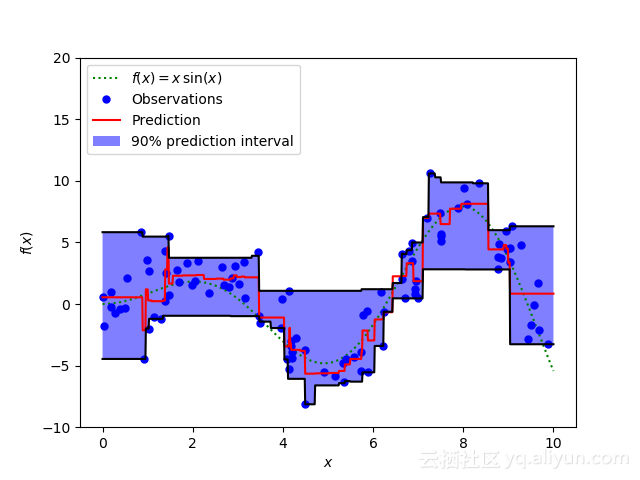﻿﻿

▌比较研究

﻿﻿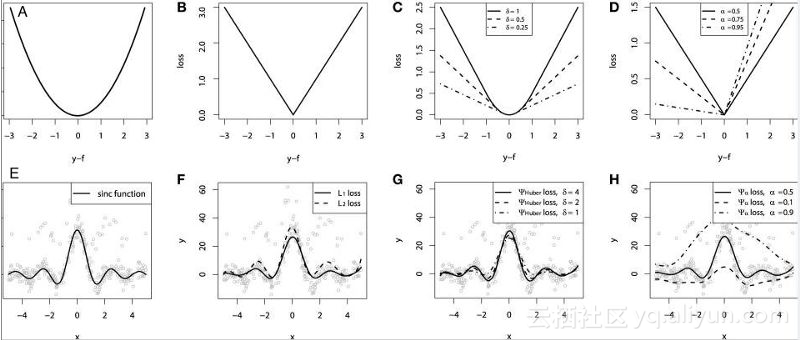Quantile Loss对相应的置信水平给出了很好的估计。

﻿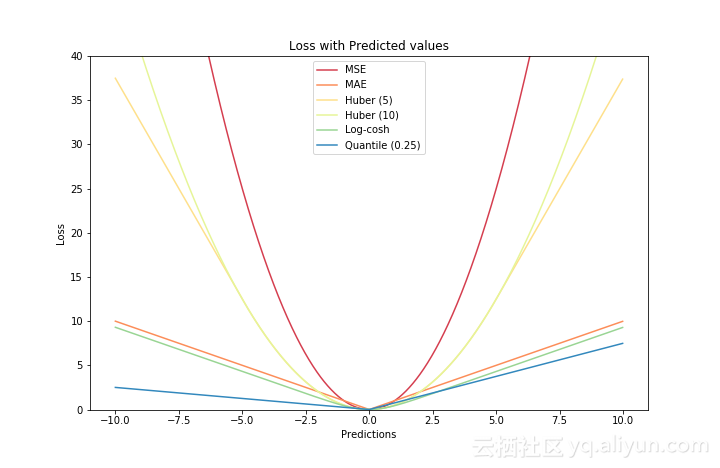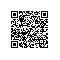使用钉钉扫一扫加入圈子
+ 订阅